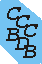Computational Chemistry Comparison and Benchmark DataBase Release 22 (May 2022) Standard Reference Database 101 National Institute of Standards and Technology Home All data for one species Geometry Experimental Calculated Comparisons Bad Calculations Tutorials and Explanations Vibrations Experimental Calculated Scale factors Reactions Entropies Ions List Ions Energy Electron Affinity Proton Affinity Ionization changes point group Experimental One molecule all properties One property a few molecules Geometry Vibrations Energy Electrostatics Reference Data Calculated Energy Optimized Reaction Internal Rotation Orbital Nuclear repulsion energy Correlation Ion Excited State Basis Set Extrapolation Geometry Vibrations Frequencies Zero point energy (ZPE) Scale Factors Bad Calculations Electrostatics Charges Dipole Quadrupole Polarizability Spin Entropy and Heat Capacity Reaction Lookup by property Comparisons Geometry Vibrations Energy Entropy Electrostatics Ion Resources Info on Results Calculations Done Basis functions used I/O files Glossary Conversion Forms Links NIST Links External links Thermochemistry Tutorials Vibrations Entropy Energy Electrostatics Geometry Cost Bad Calculations FAQ Help Units Choose Units Explanations Credits Just show me Summary Using List Recent molecules Molecules Geometry Vibrations Energy Similar molecules Ions, Dipoles, etc. Index of CCCBDB Feedback You are here: Calculated > Reaction > Transition State > IRC

# IRC

Please click on a reaction below.

 Reaction 1 H + H2 = H2 + H Reaction 2 CH4 + F = CH3 + HF Reaction 3 CH3CH2Cl = CH2CH2 + HCl Reaction 4 H + H2O = H2 + OH Reaction 5 N2O + H = N2 + OH Reaction 6 HO2 = OH + O Reaction 7 H + NH3 = H2 + NH2 Reaction 8 H + CH4 = H2 + CH3 Reaction 9 H + SiH4 = H2 + SiH3 Reaction 10 HCN = CNH Reaction 11 H + PH3 = H2 + PH2 Reaction 12 H + H2S = H2 + HS Reaction 13 OH + CH4 = H2O + CH3